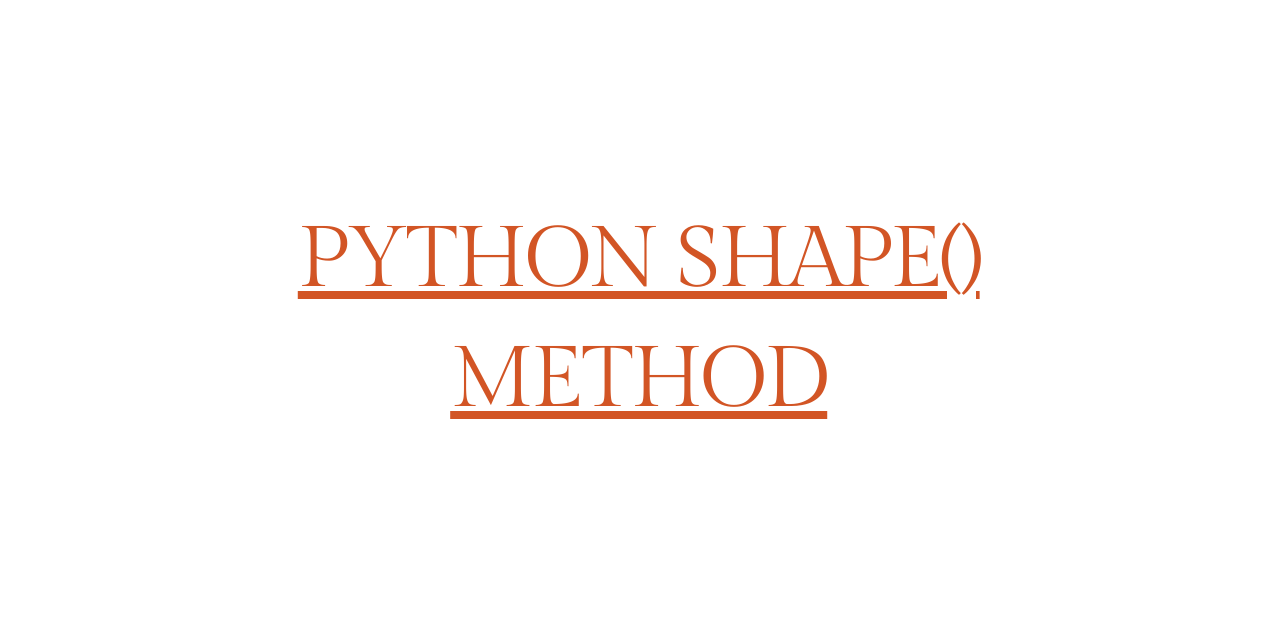# Python shape() method - All you need to know!

Published on August 3, 2022By Safa MulaniWhile we believe that this content benefits our community, we have not yet thoroughly reviewed it. If you have any suggestions for improvements, please let us know by clicking the “report an issue“ button at the bottom of the tutorial.

So, let us begin!!

## Use of Python shape() method

When it comes to the analysis of data and its variants, it is extremely important to realize the volume of data. That is, before we plan to analyze the data and perform synthesis on it, we need to be aware of the dimensions of the data.

This is when the Python shape() method comes into the picture.

With the shape() method, comes the flexibility to obtain the dimensions of any Python object. Yes, it returns a tuple value that indicates the dimensions of a Python object.

To understand the output, the tuple returned by the shape() method is the actual number of elements that represent the value of the dimension of the object.

Usually, on a broader scale, the shape() method is used to fetch the dimensions of Pandas and NumPy type objects in python.

Every value represented by the tuple corresponds to the actual dimension in terms of array or row/columns.

Let us now have a look at the variant of the same in the upcoming section.

## Variant 1: Pandas shape attribute

When we try to associate the Pandas type object with the shape method looking for the dimensions, it returns a tuple that represents rows and columns as the value of dimensions.

Syntax:

``````dataframe.shape
``````

We usually associate shape as an attribute with the Pandas dataframe to get the dimensions of the same.

Example 01:

In this example, we have created a dataframe from a Python list using DataFrame() method. Post which, we apply the dataframe.shape to check for the dimensions.

As the data that we have passed has two rows and two columns (2x2), the shape method returns us the number of rows and columns as the result.

``````import pandas as pd

data =[['P','Q'], [0, 1]]

data_frame = pd.DataFrame(data)

print(data_frame)
print("Shape of the data frame:")
print(data_frame.shape)
``````

Output:

`````` 0  1
0  P  Q
1  0  1
Shape of the data frame:
(2, 2)
``````

Example 02:

In this example, we have created an empty dataframe using DataFrame() function. Then, with the shape() method, we can get the dimensions of the empty dataframe

``````import pandas as pd

data_frame = pd.DataFrame()

print(data_frame)
print("Shape of the data frame:")
print(data_frame.shape)
``````

Output:

``````Empty DataFrame
Columns: []
Index: []
Shape of the data frame:
(0, 0)
``````

## Variant 2: NumPy shape method

With NumPy data structure, we store data elements in the form of an array. When we associate the shape() method with the NumPy array, the dimensions of the array are represented in the form of a tuple.

Syntax:

``````array.shape
``````

Example 01:

Here, we have created a NumPy array with no dimensions. Further, we have applied the shape() method on the array to get the dimensions of the created array.

``````import numpy as np

ar = np.array(0)

print(ar)
print("Shape of the array:")
print(ar.shape)
``````

Output:

``````0
Shape of the array:
()
``````

Example 02:

In this example, we have created a NumPy array and added elements to it. This is achieved using numpy.array() function. Now, we apply the shape() method to the array of elements.

``````import numpy as np

ar = np.array([[12,20] ,[13,15]])

print(ar)
print("Shape of the array:")
print(ar.shape)
``````

Output:

``````[[12 20]
[13 15]]
Shape of the array:
(2, 2)
``````

## Conclusion

By this, we have come to the end of this topic. Feel free to comment below, in case you come across any questions.

For more such posts related to Kubernetes, Stay tuned with us.

Till then, Happy Learning! :)

Thanks for learning with the DigitalOcean Community. Check out our offerings for compute, storage, networking, and managed databases.Safa Mulani

author

#### Still looking for an answer?

Ask a questionSearch for more help

Click below to sign up and get \$200 of credit to try our products over 60 days!

### Popular Topics##### Hollie's Hub for Good

Working on improving health and education, reducing inequality, and spurring economic growth? We’d like to help.##### Become a contributor

You get paid; we donate to tech nonprofits.

##### Welcome to the developer cloud

DigitalOcean makes it simple to launch in the cloud and scale up as you grow – whether you’re running one virtual machine or ten thousand.© 2023 DigitalOcean, LLC.